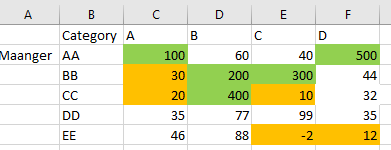Announcements
[WEBINAR] Accenture & Qlik: Accelerating BI Migration to SaaS with Qlik on Dec 13th: REGISTER
cancel
Showing results for
Did you mean:Creator

## Rank in Pivot table

Hi, We have a requirement to highlight the top 5 and bottom 5 values in the pivot table. We can use the rank function to get the top 5 but I am unable to understand how to write this function for the cross table format of the pivot table.

I have attached the screenshot of the sample data with the expected output.I searched before posting but did not get the info.

1 Solution

Accepted SolutionsMVP

You might have to use Aggr() function here

``Aggr(If(Rank(TOTAL Sum(Measure)) < 6, Green(), If(Rank(TOTAL -Sum(Measure)) < 6, Yellow())), Maanger, Category)``
6 RepliesMVP

Do you have any other dimension in pivot table?  If so how would you want to show Top5?Creator
Author

I don't have any other dimension, I just need to highlight the top5 and bottom 5 values.

in the attachment, i have highlighted the top 5 in green and bottom 5 in the amber color the same way I want to show.MVP

You might have to use Aggr() function here

``Aggr(If(Rank(TOTAL Sum(Measure)) < 6, Green(), If(Rank(TOTAL -Sum(Measure)) < 6, Yellow())), Maanger, Category)``MVP

Assuming you have two dimensions of which one is dragged to column. You can write the below expression in background color

Assuming you have Sum(Value) as your expression

=if(sum(Value)>= max(total aggr(sum(Value),Category,Name),4),green(),
if(sum(Value)<= min(total aggr(sum(Value),Category,Name),4),red()))MVP

@sunny_talwar  gotcha!!!Creator
Author

Thanks SunnyCommunity Browser